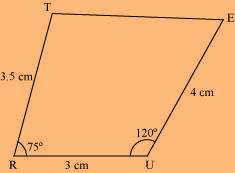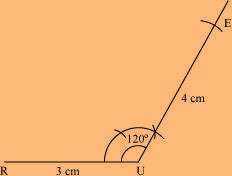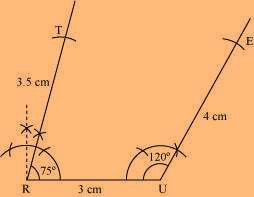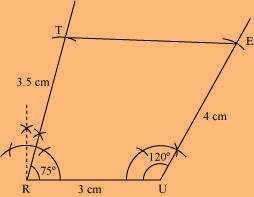# Construct the following quadrilateral: Quadrilateral TRUE TR = 3.5 cm RU = 3 cm UE = 4 cm ∠R = 75° ∠U = 120°

SolutionWe can draw the quadrilateral TRUE using the steps given below

(1) Draw a line segment RU of 3 cm and an angle of 120º at point U. As vertex E is 4 cm away from vertex U, cut a line segment UE of 4 cm from this ray.(2) Now draw an angle of 75º at point R. As vertex T is 3.5 cm away from vertex R, cut a line segment RT of 3.5 cm from this ray.(3) Join T to E.(0)(0)